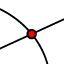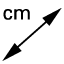# Rhombus Construction Template (V2)

## In the GeoGebra app below,

1. Construct a circle with center A that passes through B. 2. Plot a point C anywhere on the circle you just made. 3. Construct a circle with center B that passes through A. 4. Construct a circle with center C that passes through A. 5. Take a look at the circles you just constructed in steps (3) - (4), and find where they intersect. use the INTERSECTtool to plot and label a point D where these two circles intersect. 6. Use the POLYGONtool to construct quadrilateral ABDC. Keep going! Further directions appear below the GeoGebra app.

## Use the tools below to construct a rhombus. Make sure your construction always remains a rhombus (no matter where you drag its vertices)!

Use the DISTANCEtool and touch each side of quadrilateral ABDC to display its length. Then select the MOVEtool to move A, B, and or C around. What do you notice?

Given your response above, what would be the most specific name we could use to describe this quadrilateral you've just constructed?

Now use the other tools in the GeoGebra app above to construct diagonals, their intersection, and interior angle measures and side lengths of the four triangles that can be made from these diagonals. Then check as many boxes (below) that are true for this special quadrilateral.

Select all that apply
• A
• B
• C
• D
• E
• F
• G
• H
• I